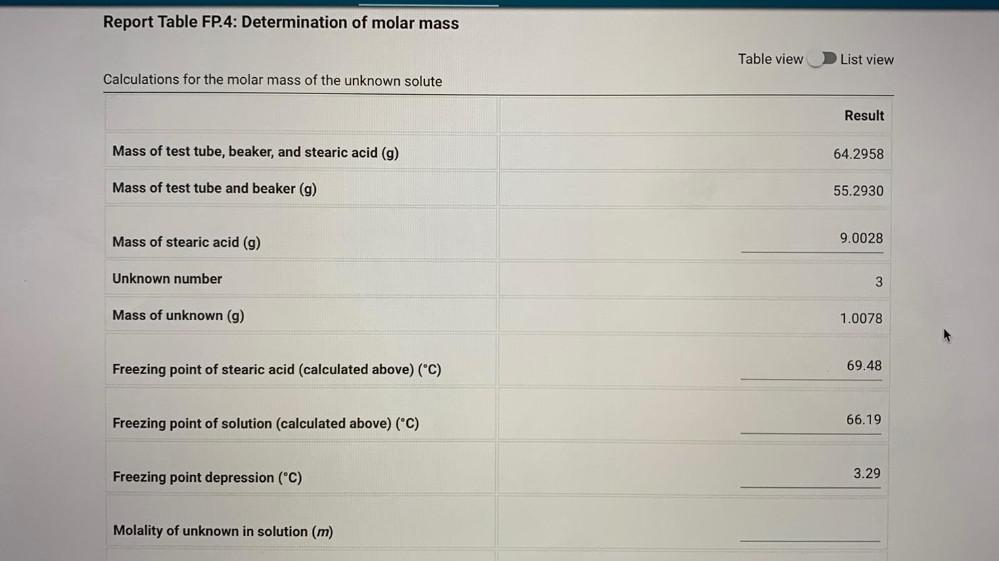Question:

# Determination of molar mass Calculations for the molar mass of the unknown solute Result Mass of tesDetermination of molar mass Calculations for the molar mass of the unknown solute Result Mass of test tube, beaker, and stearic acid (g) 64.2958 Mass of test tube and beaker (g) 55.2930 Mass of stearic acid (g) 9.0028 Unknown number 3 Mass of unknown (g) 1.0078 Freezing point of stearic acid (calculated above) (°C) 69.48 Freezing point of solution (calculated above) (°C) 66.19 Freezing point depression (°C) 3.29 Molality of unknown in solution (m)